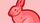cancel
Showing results for
Did you mean:

## PHP 4.4.9 without MHASH available . . .

Sorry, newbie question, but attempting to use SIM with a custom php shopping cart --  don't have MHASH on the server, and using PHP 4.4.9 . . . how does the fingerprint thing work without it?Member
1 ACCEPTED SOLUTION

Accepted Solutions

Sorry, I should have removed the bin2hex() function, you no longer need it :)

Replace:

\$fingerprint = bin2hex(mhash(MHASH_MD5, \$loginID . "^" . \$sequence . "^" . \$timeStamp . "^" . \$amount . "^", \$transactionKey));

With:

\$fingerprint = mhash(MHASH_MD5, \$loginID . "^" . \$sequence . "^" . \$timeStamp . "^" . \$amount . "^", \$transactionKey);4 REPLIES 4

You can use the following code snippet to create the mhash() function which basically does the same thing as the mhash library:

if (!function_exists('mhash'))
{
if (!defined('MHASH_MD5')) define('MHASH_MD5', 1);

function mhash(\$hash, \$data, \$key)
{
if (\$hash == MHASH_MD5)
{
// RFC 2104 HMAC implementation for php.
// Creates an md5 HMAC.
// Eliminates the need to install mhash to compute a HMAC
// Hacked by Lance Rushing

\$b = 64; // byte length for md5
if (strlen(\$key) > \$b) {
\$key = pack("H*",md5(\$key));
}

}
}
}Member

Many, many thanks.  I am, however, running into a 99 error now, I've been changing variables left and right but to no avail.  Any thoughts?  Code below:

<?php

// the parameters for the payment can be configured here
// the API Login ID and Transaction Key must be replaced with valid values
\$transactionKey = "developer testtransaction Key";

//\$proc_final_total is an XX.XX formatted number determined earlier on the page.

\$amount         = \$proc_final_total;
\$description     = "Sample Transaction";
\$label             = "Submit Payment"; // The is the label on the 'submit' button
\$testMode        = "false";
\$url            = "https://test.authorize.net/gateway/transact.dll";

// If an amount or description were posted to this page, the defaults are overidden
if (array_key_exists("amount",\$_REQUEST))
{ \$amount = \$_REQUEST["amount"]; }
if (array_key_exists("amount",\$_REQUEST))
{ \$description = \$_REQUEST["description"]; }

// an invoice is generated using the date and time
\$invoice    = date(YmdHis);
// a sequence number is randomly generated
\$sequence    = rand(1, 1000);
// a timestamp is generated
\$timeStamp    = time();

if (!function_exists('mhash'))
{
if (!defined('MHASH_MD5')) define('MHASH_MD5', 1);

function mhash(\$hash, \$data, \$key)
{
if (\$hash == MHASH_MD5)
{
// RFC 2104 HMAC implementation for php.
// Creates an md5 HMAC.
// Eliminates the need to install mhash to compute a HMAC
// Hacked by Lance Rushing

\$b = 64; // byte length for md5
if (strlen(\$key) > \$b) {
\$key = pack("H*",md5(\$key));
}

}
}
}
\$fingerprint = bin2hex(mhash(MHASH_MD5, \$loginID . "^" . \$sequence . "^" . \$timeStamp . "^" . \$amount . "^", \$transactionKey));
?>
<form method='post' action='<?php echo \$url; ?>' >
<!--  Additional fields can be added here as outlined in the SIM integration
guide at: http://developer.authorize.net -->
<input type='hidden' name='x_amount' value='<?php echo \$amount; ?>' />
<input type='hidden' name='x_description' value='<?php echo \$description; ?>' />
<input type='hidden' name='x_invoice_num' value='<?php echo \$invoice; ?>' />
<input type='hidden' name='x_fp_sequence' value='<?php echo \$sequence; ?>' />
<input type='hidden' name='x_fp_timestamp' value='<?php echo \$timeStamp; ?>' />
<input type='hidden' name='x_fp_hash' value='<?php echo \$fingerprint; ?>' />
<input type='hidden' name='x_test_request' value='<?php echo \$testMode; ?>' />
<input type='hidden' name='x_show_form' value='PAYMENT_FORM' />
<input type='submit' value='<?php echo \$label; ?>' />
</form>Sorry, I should have removed the bin2hex() function, you no longer need it :)

Replace:

\$fingerprint = bin2hex(mhash(MHASH_MD5, \$loginID . "^" . \$sequence . "^" . \$timeStamp . "^" . \$amount . "^", \$transactionKey));

With:

\$fingerprint = mhash(MHASH_MD5, \$loginID . "^" . \$sequence . "^" . \$timeStamp . "^" . \$amount . "^", \$transactionKey);